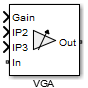# VGA

Variable gain amplifier

## Library

Elements## Description

The VGA block models a RF Blockset™ variable gain amplifier. The Simulink® signal controls the non-linear gain. The `VGA` output voltage is a function of the input voltage:

`${V}_{out}=g*{V}_{in}+{c}_{2}*{V}_{in}{}^{2}+{c}_{3}*{V}_{in}{}^{2}$`

The table shows the formulas for the coefficients, g, c2, and c3

Coefficient Formulae

Input ReferredOutput Referred
g

`$g=\frac{2\sqrt{G*{Z}_{out}*{Z}_{in}}}{|{Z}_{in}|}$`

c2

`${C}_{2}=\frac{g}{\sqrt{2*IP2*{Z}_{in}}}volt{s}^{-1}$`

`${C}_{2}=\frac{{g}^{2}}{\sqrt{8*IP2*{Z}_{out}}}volt{s}^{-1}$`

c3

`${C}_{3}=\frac{4}{3}.\frac{g}{2*IP3*{Z}_{in}}volt{s}^{-2}$`

`${C}_{2}=\frac{4}{3}.\frac{{g}^{3}}{8*IP3*{Z}_{out}}volt{s}^{-2}$`

The three Simulink input ports are `Gain`, `IP2` (second-order intercept point), and `IP3` (third-order intercept point). `In` is the RF Blockset voltage input and `OUT` is RF Blockset voltage output.

## Parameters

Input impedance (Ohm)

Input impedance of the amplifier, specified as a scalar. The default value is `50`  ohms.

Output impedance (Ohm)

Output impedance of the amplifier, specified as a scalar. The default value is `50`  ohms.

Intercept points convention

Input-referred or output-referred convention for IP2 or IP3 intercept points, specified as `Input` or `Output`.

The default is `Output`.

Ground and hide negative terminals

Select this option to internally ground and hide the negative terminals. Clear the option to expose the negative terminals. By exposing these terminals, you can connect them to other parts of your model.

By default, this option is selected.